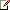# Range Object

 Excel Developer Reference

Represents a cell, a row, a column, a selection of cells containing one or more contiguous blocks of cells, or a 3-D range.

Remarks

The following properties and methods for returning a Range object are described in the examples section:

Example

Use Range(

arg

), where

arg

names the range, to return a Range object that represents a single cell or a range of cells. The following example places the value of cell A1 in cell A5.

Visual Basic for Applications
```  ```Worksheets("Sheet1").Range("A5").Value = _
Worksheets("Sheet1").Range("A1").Value```
```

The following example fills the range A1:H8 with random numbers by setting the formula for each cell in the range. When it’s used without an object qualifier (an object to the left of the period), the Range property returns a range on the active sheet. If the active sheet isn’t a worksheet, the method fails. Use the Activate method to activate a worksheet before you use the Range property without an explicit object qualifier.

Visual Basic for Applications
```  ```Worksheets("Sheet1").Activate
Range("A1:H8").Formula = "=Rand()"    'Range is on the active sheet```
```

The following example clears the contents of the range named Criteria.Note
If you use a text argument for the range address, you must specify the address in A1-style notation (you cannot use R1C1-style notation).
Visual Basic for Applications
```  `Worksheets(1).Range("Criteria").ClearContents`
```

Use Cells(

row

,

column

) where

row

is the row index and

column

is the column index, to return a single cell. The following example sets the value of cell A1 to 24.

Visual Basic for Applications
```  `Worksheets(1).Cells(1, 1).Value = 24`
```

The following example sets the formula for cell A2.

Visual Basic for Applications
```  `ActiveSheet.Cells(2, 1).Formula = "=Sum(B1:B5)"`
```

Although you can also use `Range("A1")` to return cell A1, there may be times when the Cells property is more convenient because you can use a variable for the row or column. The following example creates column and row headings on Sheet1. Notice that after the worksheet has been activated, the Cells property can be used without an explicit sheet declaration (it returns a cell on the active sheet).Note
Although you could use Visual Basic string functions to alter A1-style references, it's much easier (and much better programming practice) to use the `Cells(1, 1)` notation.
Visual Basic for Applications
```  ```Sub SetUpTable()
Worksheets("Sheet1").Activate
For TheYear = 1 To 5
Cells(1, TheYear + 1).Value = 1990 + TheYear
Next TheYear
For TheQuarter = 1 To 4
Cells(TheQuarter + 1, 1).Value = "Q" & TheQuarter
Next TheQuarter
End Sub```
```

Use

expression

.Cells(

row

,

column

) , where

expression

is an expression that returns a Range object, and

row

and

column

are relative to the upper-left corner of the range, to return part of a range. The following example sets the formula for cell C5.

Visual Basic for Applications
```  `Worksheets(1).Range("C5:C10").Cells(1, 1).Formula = "=Rand()"`
```

Use Range(

cell1, cell2

), where

cell1

and

cell2

are Range objects that specify the start and end cells, to return a Range object. The following example sets the border line style for cells A1:J10.Note
Notice the period in front of each occurrence of the Cells property. The period is required if the result of the preceding With statement is to be applied to the Cells property — in this case, to indicate that the cells are on worksheet one (without the period, the Cells property would return cells on the active sheet).
Visual Basic for Applications
```  ```With Worksheets(1)
.Range(.Cells(1, 1), _
.Cells(10, 10)).Borders.LineStyle = xlThick
End With```
```

Use Offset(

row, column

), where

row

and

column

are the row and column offsets, to return a range at a specified offset to another range. The following example selects the cell three rows down from and one column to the right of the cell in the upper-left corner of the current selection. You cannot select a cell that isn’t on the active sheet, so you must first activate the worksheet.

Visual Basic for Applications
```  ```Worksheets("Sheet1").Activate
'Can't select unless the sheet is active
Selection.Offset(3, 1).Range("A1").Select```
```

Use Union(

range1, range2

, ...) to return multiple-area ranges — that is, ranges composed of two or more contiguous blocks of cells. The following example creates an object defined as the union of ranges A1:B2 and C3:D4, and then selects the defined range.

Visual Basic for Applications
```  ```Dim r1 As Range, r2 As Range, myMultiAreaRange As Range
Worksheets("sheet1").Activate
Set r1 = Range("A1:B2")
Set r2 = Range("C3:D4")
Set myMultiAreaRange = Union(r1, r2)
myMultiAreaRange.Select```
```

If you work with selections that contain more than one area, the Areas property is very useful. It divides a multiple-area selection into individual Range objects and then returns the objects as a collection. You can use the Count property on the returned collection to check for a selection that contains more than one area, as shown in the following example.

Visual Basic for Applications
```  ```Sub NoMultiAreaSelection()
NumberOfSelectedAreas = Selection.Areas.Count
If NumberOfSelectedAreas > 1 Then
MsgBox "You cannot carry out this command " & _
"on multi-area selections"
End If
End Sub```
```

Reference
Using Defined Names